C Program to Find Total Number of Digit in a Given Number

In this tutorial you will learn about the C Program to Find Total Number of Digit in a Given Number and its application with practical example.

C Program to Find Total Number of Digit in a Given Number.

In this tutorial, we will learn to create a C program that will Find the Total Number of digits in a Given Number in C programming.

Prerequisites

Before starting with this tutorial we assume that you are best aware of the following C programming topics:

• Operators in C Programming.
• Basic Input and Output function in C Programming.
• Basic C programming.
• While loop in c programming.

Finding Total Number of Digits in a Given Number.

As we all know the c programming is a very powerful language. In the c programming language, we have many pre-defined functions for arithmetic operations. but today we will count the number of digits in a number with the help of a while loop.

With the help of this program, we can Find the Total Number of digits in a Given Number.

Output:-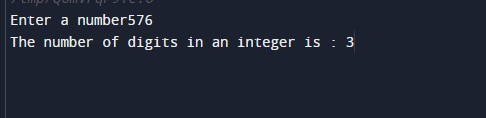In the above program, we have first initialized the required variable.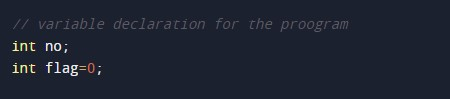• flag= it will hold the integer value for numbers.
• no = it will hold the integer value of number input.

Display message to Take input number from the user.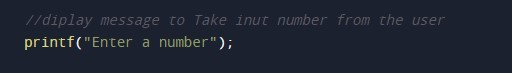Scanning the input number and storing it to a variable.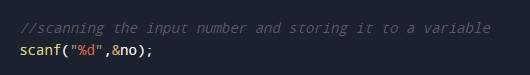While loop to get the number of digits in a number.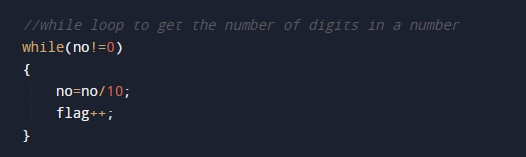Printing output of the program.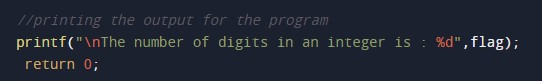In this tutorial we have learn about the C Program to Find Total Number of Digit in a Given Number and its application with practical example. I hope you will like this tutorial.